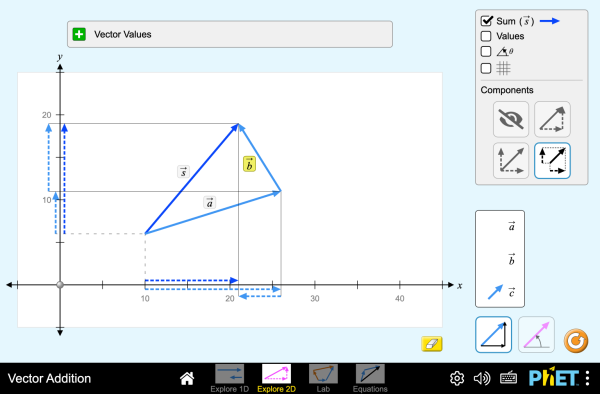下載嵌入 關閉 嵌入模擬教學執行複本 利用 HTML 來嵌入模擬教學執行複本。您可以在 HTML 中改變已嵌入模擬教學的寬度及高度。 嵌入啓動模擬教學之圖樣原始模擬教學與翻譯

### 標題

• Vectors
• Vector Components
• Equations

### 學習目標

• ●解釋甚麼是向量
• ●向量的角度形式和分量形式之間的轉換

### 標準對齊

#### 共用核心 - 數學

HSN-VM.A.1
(+) Recognize vector quantities as having both magnitude and direction. Represent vector quantities by directed line segments, and use appropriate symbols for vectors and their magnitudes (e.g., v, |v|, ||v||, v).
HSN-VM.A.2
(+) Find the components of a vector by subtracting the coordinates of an initial point from the coordinates of a terminal point.
HSN-VM.B.4
HSN-VM.B.4a
Add vectors end-to-end, component-wise, and by the parallelogram rule. Understand that the magnitude of a sum of two vectors is typically not the sum of the magnitudes.
HSN-VM.B.4b
Given two vectors in magnitude and direction form, determine the magnitude and direction of their sum.
HSN-VM.B.4c
Understand vector subtraction v - w as v + (-w), where -w is the additive inverse of w, with the same magnitude as w and pointing in the opposite direction. Represent vector subtraction graphically by connecting the tips in the appropriate order, and perform vector subtraction component-wise.
HSN-VM.B.5
(+) Multiply a vector by a scalar.
HSN-VM.B.5a
Represent scalar multiplication graphically by scaling vectors and possibly reversing their direction; perform scalar multiplication component-wise, e.g., as c(vx, vy) = (cvx, cvy).
HSN-VM.B.5b
Compute the magnitude of a scalar multiple cv using ||cv|| = |c|v. Compute the direction of cv knowing that when |c|v ≠ 0, the direction of cv is either along v (for c > 0) or against v (for c < 0).

### 教學提示模擬教學的控制概觀、模型簡化與引導學生思考 ( PDF ).

### 老師提供的活動

Clases Demostrativas Interactivas (ILD) de VectoresDiana López 大學-簡介

Concept questions for Physics using PhET (Inquiry Based)Trish Loeblein 高中

Algebra-based Physics Semester one lessons, clicker questions, and schedule in pdf (Inquiry Based)Trish Loeblein 大學-簡介

Vector Addition 1: Introduction to vector math (Inquiry Based)Trish Loeblein 大學-簡介

Vector Addition 2: Understanding Force equilibrium (Inquiry Based)Trish Loeblein 高中

SECUNDARIA: Alineación PhET con programas de la SEP México (2011 y 2017)Diana López 高中

PREPARATORIA: Alineación de PhET con programas de la DGB México (2017)Diana López 大學-簡介

Figuras y cuerpos geométricos. Andrés Gualberto Palomo Cuevas 高中 Remote

Guia de aprendizaje sobre vectores en simuladores jorge luis rodriguez acosta 國中 指引 數學
Reforzamiento de vectores Andrés Gualberto Palomo Cuevas 高中 實驗室 物理
Adding Vectors Visually and Mathematically - Intro Jeremy smith 高中

Remote

equilibrium of forces tahani sarayreh 高中

Remote

Forces at equilibrium Nawal Nayfeh 大學-簡介

Remote

Find The components of a vector Dr Akhlaq Ahmad 高中 實驗室

Soma de Vetores Juciane1 Matrtins 高中 實驗室 物理

Norwegian Bokmal 全部 Norsk bokmålVektorsum
Serbian 全部 СрпскиСабирање вектора

HTML5 sims can run on iPads and Chromebooks, as well as PC, Mac, and Linux systems.

iOS 12+ Safari

Android:
Not officially supported. If you are using the HTML5 sims on Android, we recommend using the latest version of Google Chrome.

Chromebook:
The HTML5 and Flash PhET sims are supported on all Chromebooks.
Chromebook compatible sims

Windows Systems:

Macintosh Systems:

Linux Systems:

• Brandon Li (developer)
• Chris Malley (developer)
• Martin Veillette (developer)
• Michael Dubson (original designer)
• Diana López Tavares
• Amanda McGarry
• Ariel Paul
• Kathy Perkins
• almond-0.2.9.js
• base64-js-1.2.0.js
• FileSaver-b8054a2.js
• font-awesome-4.5.0
• game-up-camera-1.0.0.js
• he-1.1.1.js
• himalaya-0.2.7.js
• jama-1.0.2
• jquery-2.1.0.js
• lodash-4.17.4.js
• pegjs-0.7.0.js
• seedrandom-2.4.2.js
• text-2.0.12.js
• TextEncoderLite-3c9f6f0.js